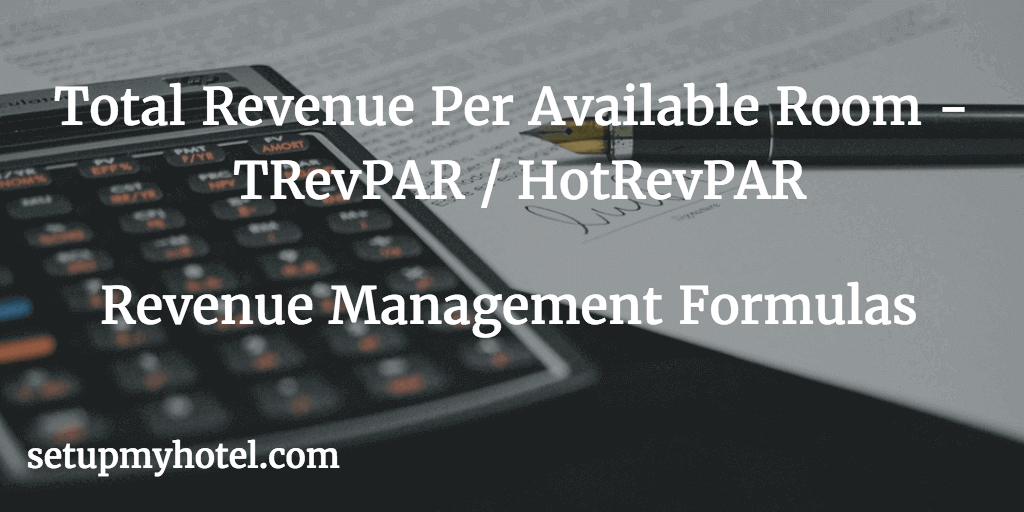# HotRevPAR / TRevPAR (Total Revenue Per Available Room) Online Calculator

A measure to calculate the total revenue per available room. TrevPAR calculated by the sum of room revenue, food and beverage (F&B) revenue, and other revenue divided by total available rooms.

## Calculate Total Revenue Per Availalbe Room (TRevPAR or HotRevPAR)

Total Revenue (Room + F&B + Others) :

Total Available Rooms For Sale:

## Total Revenue Per Availalbe Room (TRevPAR):

Example 1:

Total Hotel Revenue for 01st Sep 2017    = 25,585.26

Total Rooms Available for 01st Sep 2017  = 258

TRevPAR = 25,585.26 / 258

= 99.16

Example 2:

Total Hotel Revenue for 30th Aug 2017   =  33,890.00

Total Rooms Available for 30th Aug 2017 = 257

TRevPAR = 33,890.00 / 225

= 131.86

Example 3 - Monthy TRevPAR:

Total Hotel Revenue for August 2017   =  775,090.00

Total Rooms Available for August 2017 = 16410

TRevPAR = 775,090.00 / 6820

= 113.64